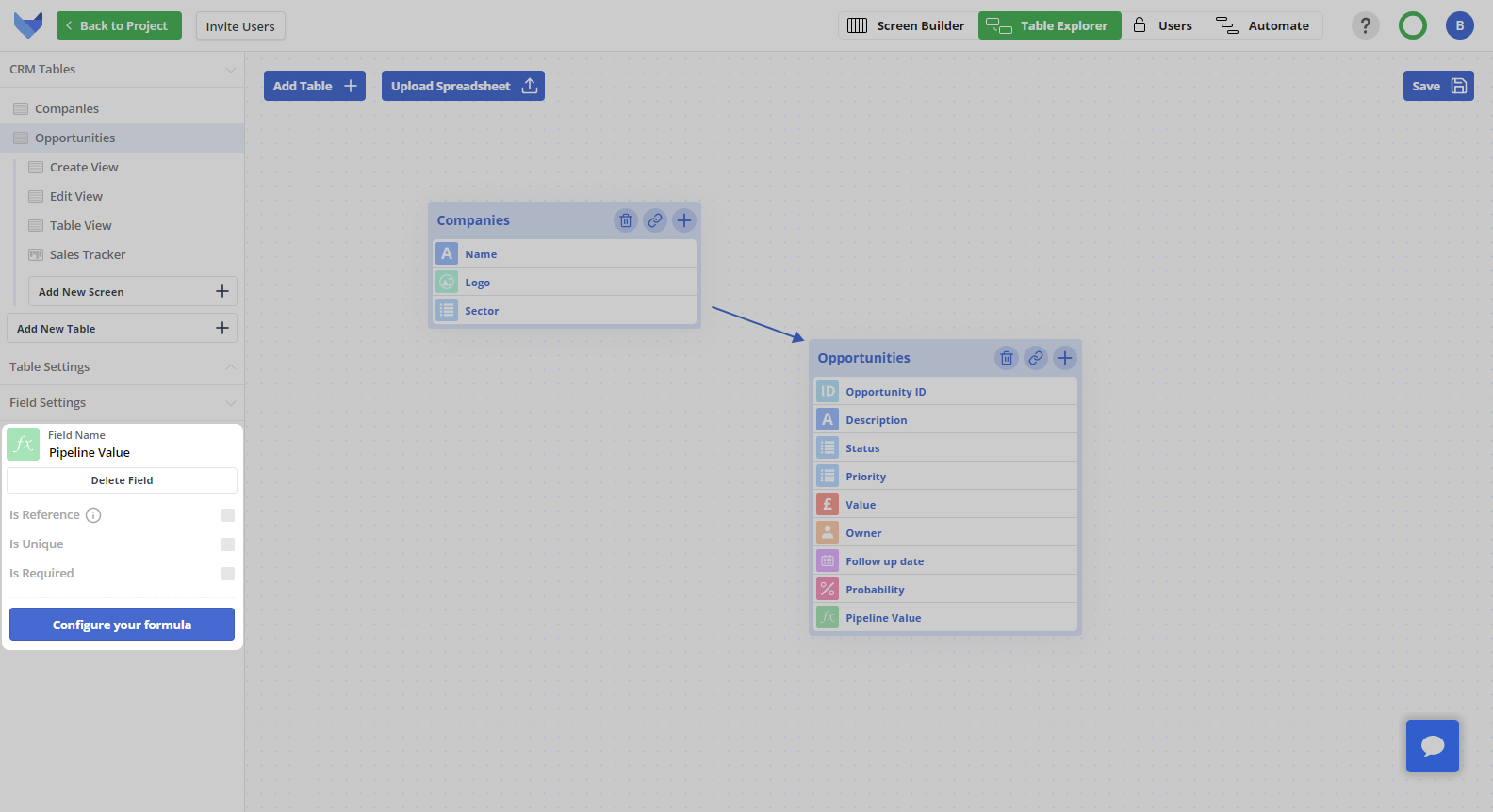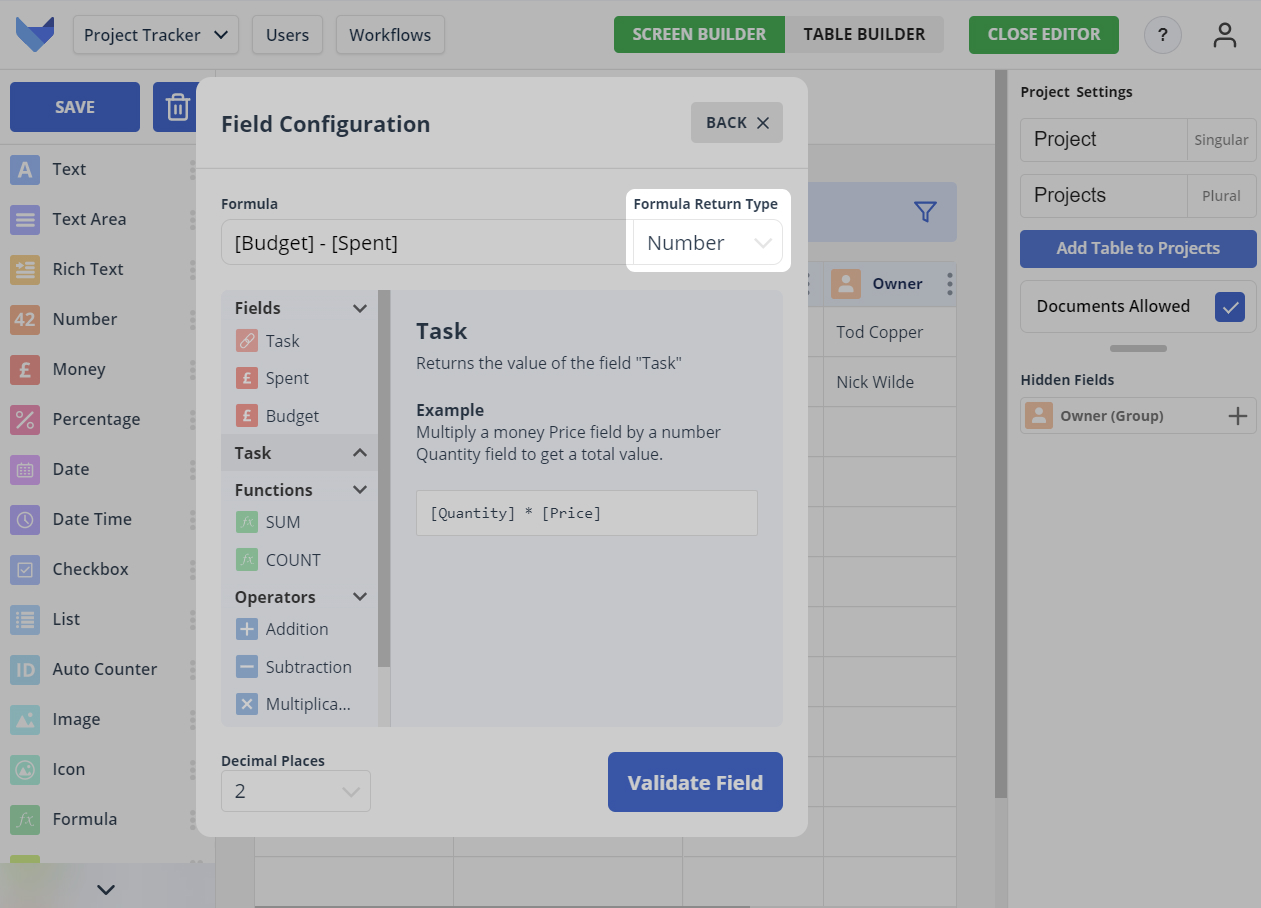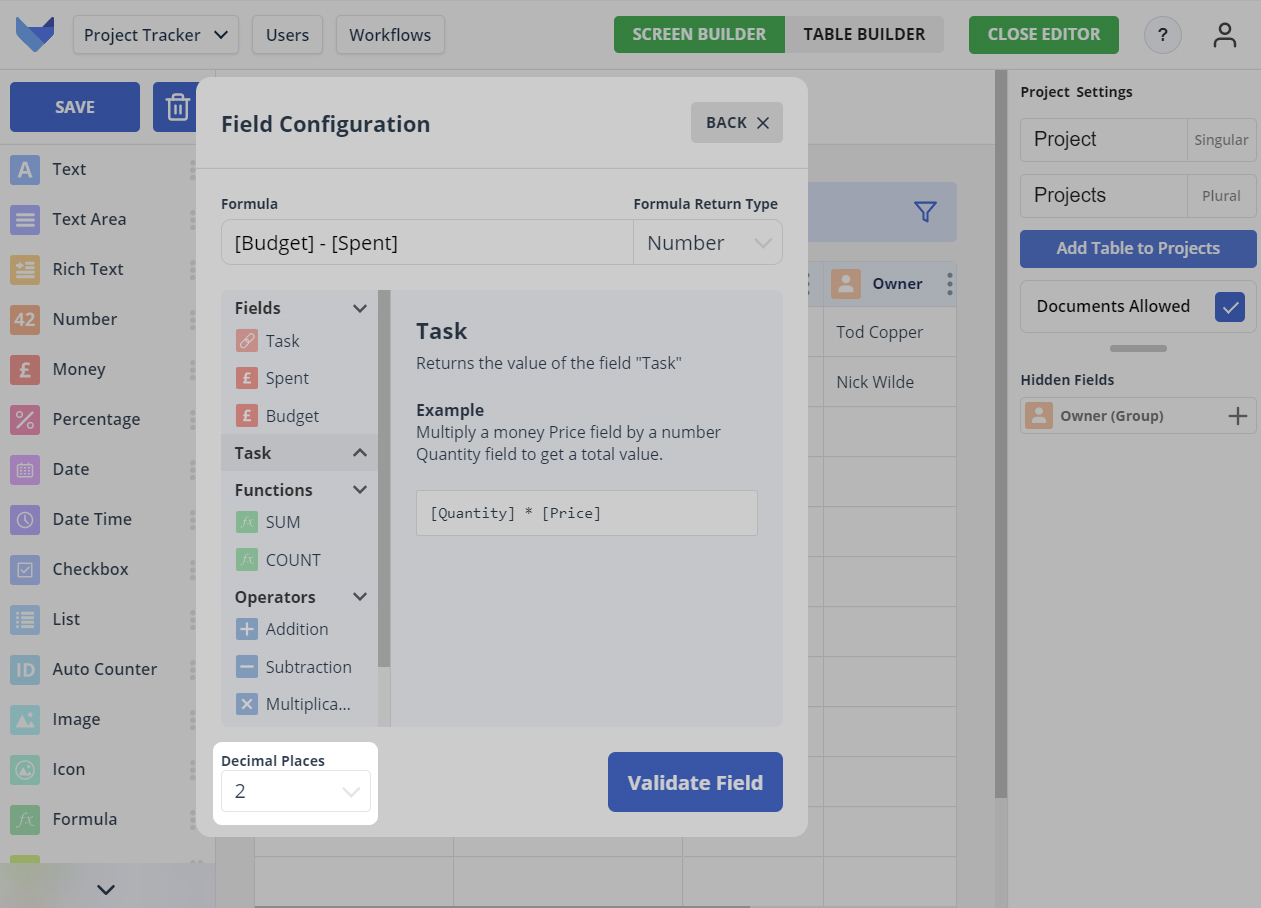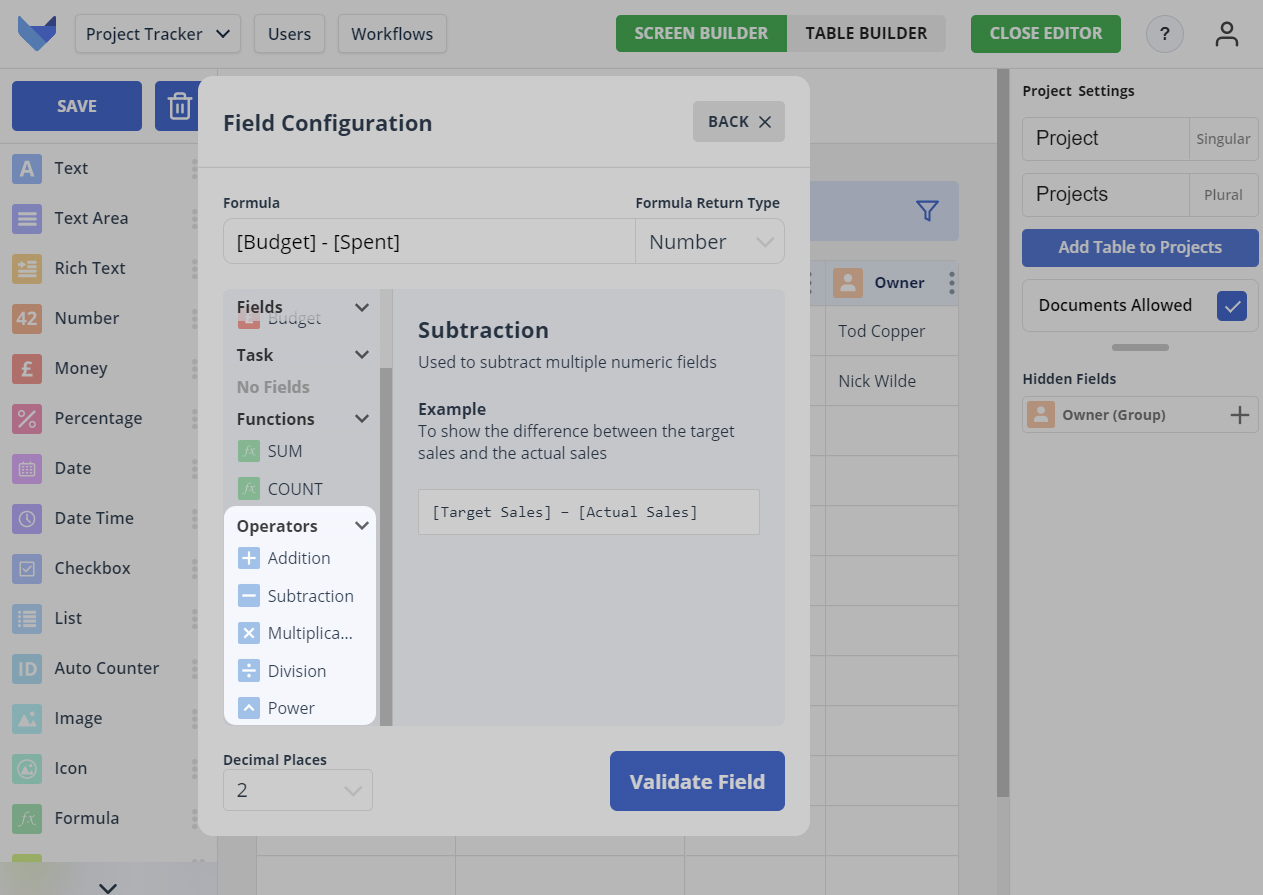# Formula Fields

Formula Fields can be used to perform calculations on data within other Fields in your Tables.

You can use basic arithmetic as well as more complex operations such as summing the total value in a Field on related child Records.

## Configuring a Formula Field

To configure the formula for a Formula Field, you will need to open the Field Settings Menu. This can be done in either the Grid View Editor or the Table Explorer.

Click on the Field and the settings will open in the left hand side panel.Click Configure your formula to show the formula configuration options.

You can enter the required formula. You can choose to type out the formula using the appropriate syntax, or use the menu of Fields, Operators and Functions. An explanation of the available Fields, Operators and Functions is available further down this page.

Confirm the formula is valid by clicking Validate Field and then click Save to confirm the new formula.

## Formula Return Types

Each formula must have a return type specified. The return type specifies the format of the output of the formula field.

The following return types are supported:

1. Number

Formula is calculated and shown as a number

2. Money

Formula is calculated and shown to 2 decimal places as a currency value for your chosen currency, where your currency options are: GBP, USD, EUR

You can change the currency of an existing formula, but note that this will not perform any currency conversion. The value will remain unchanged.

3. Percentage

Formula is calculated and converted to a percentage value### Specifying Decimal Places

For the number and money return types, you can specify the number of decimal places that should be shown.

The value calculated using the formula will be rounded to the specified number of decimal places.## Arithmetic in Formulas

To perform arithmetic operations in your Formula Field, you can use any of the following:

• ’+’ for addition
• ’-‘ for subtraction
• ‘*’ for multiplication
• ’/’ for division
• ’^’ for exponentiation, i.e. to take a value to the power of another value

Standard mathematical rules are used to determine the order of arithmetic operations.

NOTE

Ensure there is a space either side of the operation e.g. ‘1 + 2’ would be considered valid, but ‘1+2’ would not.## Referencing Fields in Formulas

To include another Field in the formula, enclose the Field Name in square brackets.

For example, if you had an Order Table with a field called ‘Quantity’ and a field called ‘Price’, you could create a formula for the total value of the Order by entering `[Quantity] * [Price]`.

You can reference Fields from linked Parent Tables using the syntax ‘ParentTableSingularName.FieldName’, ensuring you enclose this within square brackets.

For example, if you had a Project Phase Table which had a parent Project Table, assuming each Phase gets a given percentage of the Project Budget, you could create a formula for the Phase Budget by entering `[Phase Percentage] * [Project.Budget]`.

## SUM and COUNT Functions

You can use the SUM and COUNT functions to include information from linked Child Tables.

The COUNT function allows you to include the total number of related Child Records linked to a Parent. The syntax is ‘COUNT([ChildTableSingularName])’.

For example, if you had a Company Table with a child Contact Table, you could show the total number of contacts at a Company by adding a Company formula field for `COUNT([Contact])`.

The SUM function allows you to sum the value in a Field on related Child Records linked to a Parent. The syntax is ‘SUM([ChildTableSingularName.FieldName])’.

For example, if you had a Project Table with a child Task Table where Tasks have a Cost, you could show the total cost of all Tasks associated with a Project by adding a Project formula field for `SUM([Task.Cost])`.

TIP

COUNT and SUM functions can be used in conjunction with other operations in a formula.

NOTE

Ensure that you enter `COUNT` and `SUM` in block capitals. The formula is case sensitive.

### WHERE Conditions

For both the SUM and the COUNT function, you can limit the Records that are included in the summing or counting by adding a WHERE clause to your formula.

The WHERE clause allows you to filter the Records to include based on the value in a List Field on the Child Table. You can choose to count or sum all Records where the List Field matches a certain List Value, or where the List Field does not match a certain List Value.

The syntax to use is as follows:

• For counting the number of Records where the value in a list field matches a specified value:

COUNT([ChildTableSingularName] WHERE [ListFieldName] = ‘ListFieldValue’)

• For counting the number of Records where the value in a list field does not match a specified value:

COUNT([ChildTableSingularName] WHERE [ListFieldName] != ‘ListFieldValue’)

• For summing the value in a Field where the value in a list field on Records matches a specified value:

SUM([ChildTableSingularName.FieldName] WHERE [ListFieldName] = ‘ListFieldValue’)

• For summing the value in a Field where the value in a list field on Records does not match a specified value:

SUM([ChildTableSingularName.FieldName] WHERE [ListFieldName] != ‘ListFieldValue’)

For example, if you would like to know the number of Tasks related to a Project that are not yet completed, you could add a formula field for `COUNT([Tasks] WHERE Status != 'Done')`.

## Calculating Formula Fields

Formula values are calculated as Records are viewed and so will always show the latest information.

NOTE

Blank values in Fields are treated as 0 for formula fields.